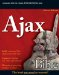Operating on Your Data with Operators

You’ve already seen that you can use + to add two numbers together:

function adder(operand1, operand2) {   return operand1 + operand2; }

The + here is a JavaScript operator, and you use operators to work on your data. There are many JavaScript operators besides +; for example, you can use * to multiply two numbers together:

function multiplier(operand1, operand2) {   return operand1 * operand2; }

The addition operator is +, the multiplication operator is *, the subtraction operator is -, and the division operator is /. What other operators are available? You can see them all in Table 2.1. (Don’t try to memorize what you see there. Come back to this table throughout the book as needed.)

Table 2.1: JavaScript OperatorsOpen table as spreadsheet

Operator

Description

Arithmetic Operators

+

++

Increments the value in a variable by one.

-

Subtracts one number from another. Also can change the sign of its operand like this: -variableName.

--

Decrements the value in a variable by one.

*

Multiplies two numbers.

/

Divides two numbers.

%

Evaluates to the remainder after dividing two numbers using integer division.

String Operators

+

Joins (concatenates) two strings.

+=

Joins (concatenates) two strings and assigns the joined string to the first operand.

Logical Operators

&&

Evaluates to true if both operands are true; otherwise, evaluates to false.

||

Evaluates to true if either operand is true. However, if both operands are false, evaluates to false.

!

Evaluates to false if its operand is true, true if its operand is false.

Bitwise Operators

&

Sets a 1 in each bit position of both operands.

^

Sets a 1 in a bit position if the bits of one operand, but not both operands, are one.

|

Sets a 1 in a bit if either operand has a 1 in that position.

~

Flips each bit.

<<

Shifts the bits of its first operand to the left by the number of places specified by the second operand.

>>

Shifts the bits of the first operand to the right by the number of places specified by the second operand.

>>>

Shifts the bits of the first operand to the right by the number of places specified by the second operand, and shifting in zeros from the left. continued

Assignment Operators

=

Assigns the value of the second operand to the first operand.

+=

Adds two operands and assigns the result to the first operand.

-=

Subtracts two operands and assigns the result to the first operand.

*=

Multiplies two operands and assigns the result to the first operand.

/=

Divides two operands and assigns the result to the first operand.

%=

Calculates the modulus of two operands and assigns the result to the first operand.

&=

Performs a bitwise AND operation on two operands and assigns the result to the first operand.

^=

Performs a bitwise exclusive OR operation on two operands and assigns the result to the first operand.

|=

Performs a bitwise OR operation on two operands and assigns the result to the first operand.

<<=

Performs a left shift operation on two operands and assigns the result to the first operand.

>>=

Performs a sign-propagating right shift operation on two operands and assigns the result to the first operand.

>>>=

Performs a zero-fill right shift operation on two operands and assigns the result to the first operand.

Comparison Operators

==

Evaluates to true if the two operands are equal to each other.

!=

Evaluates to true if the two operands are not equal to each other.

===

Evaluates to true if the two operands are both equal and of the same type.

!==

Evaluates to true if the two operands are either not equal and/or not of the same type.

>

Evaluates to true if the first operand’s value is greater than the second operand’s value.

>=

Evaluates to true if the first operand’s value is greater than or equal to the second operand’s value.

<

Evaluates to true if the first operand’s value is less than the second operand’s value.

<=

Less-than-or-equal-to operator. Evaluates to true if the first operand’s value is less than or equal to the second operand’s value.

Special Operators

?:

Performs an “if...else” test.

,

Evaluates two expressions and returns the result of evaluating the second expression.

delete

Deletes an object and removes it from memory, or deletes an object’s property, or deletes an element in an array.

function

Creates an anonymous function. (See Chapter 3.)

in

Evaluates to true if the property you’re testing is supported by a specific object.

instanceof

Evaluates to true if the given object is an instance of the specified type.

new

Creates a new object from the specified object type.

typeof

Evaluates to the name of the type of the operand.

Void

Allows evaluation of an expression without returning any value.

You can use combination operators, like +=, to perform both an operation and to assign the results of that operation to a variable, all in one step. For example, you could do this to add 5 to the value in temperature:

var temperature; temperature = 72; temperature = temperature + 5;

But you can also use the shortcut operator += to do the addition and assignment together, like this:

var temperature; temperature = 72; temperature += 5;

Similarly, there are -=, *=, and other combination operators.

What about the ==, >, <, <=, operators in Table 2.1? You use them to make decisions in JavaScript, executing some code if a particular condition is true and other code if that condition is false, which is discussed next.Ajax Bible
ISBN: 0470102632
EAN: 2147483647
Year: 2004
Pages: 169

Similar book on Amazon